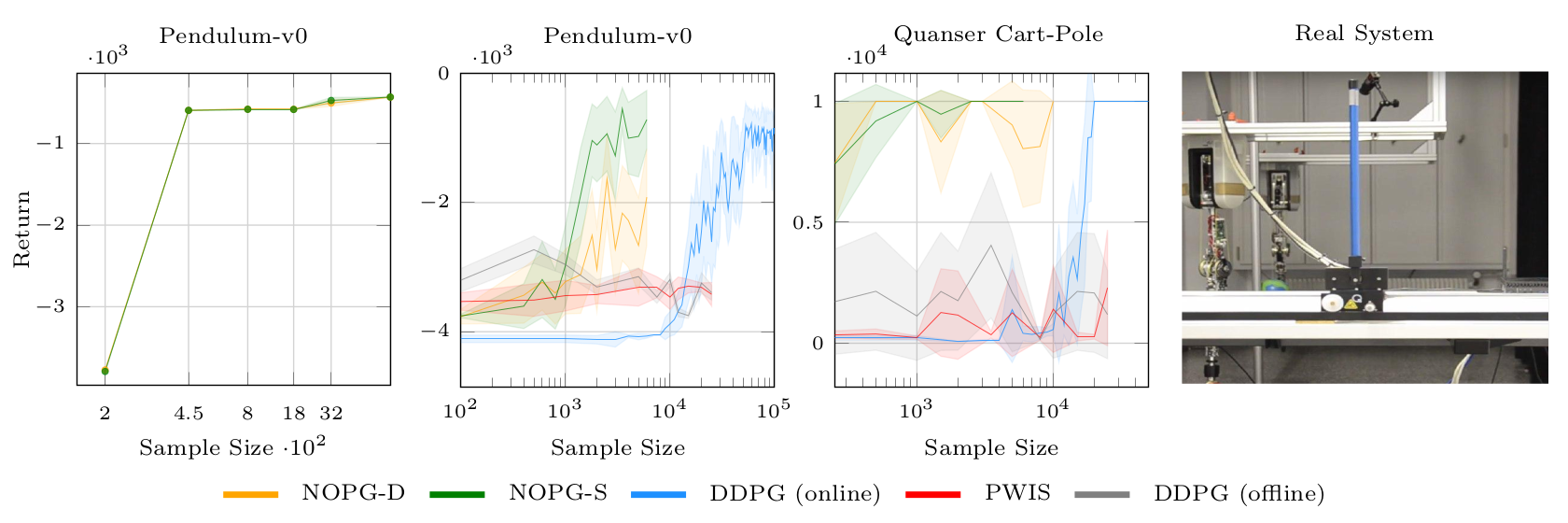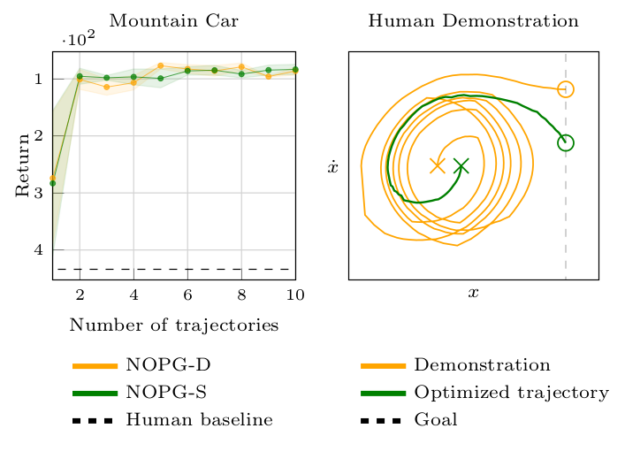# Enhancing Sample Efficiency in Reinforcement Learning with Nonparametric MethodsRecent developments in artificial intelligence and autonomous learning have shown impressive results in tasks like board games and computer games. However, the applicability of learning techniques remains mainly limited to simulated environments.

One of the major causes of this inapplicability to real-world scenarios is the general sample-inefficiency and inability to guarantee the safe operation of state-of-the-art reinforcement learning. In reinforcement learning theory, you want to improve an agent’s behavior according to a specific metric. To improve with respect to this metric, the agent can interact with the environment, from which it collects observations and rewards. Improvements can be performed in two distinct ways: on-policy and off-policy.

In the on-policy case, the improvement must be carried out by the direct interaction of the agent with the environment. This kind of improvement is mathematically simple but hinders sample efficiency as it disallows the reuse of samples. When the agent behavior is improved, the agent must re-interact with the environment to generate new on-policy samples. In the early stages of learning, the agent might be not suited for direct interaction with a physical environment, for example, because its behavior is random. In simulated tasks, there is unlimited availability of samples and there is no danger in the application of harmful behavior. However, for real-world applications, these issues are severe.

In the off-policy case, you can improve the agent’s behavior by interactions with the environment completed by other agents. This allows sample reuse and safer interaction, as the agents interacting with the environment can be experts. For example, a human could collect samples by moving a robotic arm.

The downside of off-policy improvement is the difficulty of obtaining a reliable estimation. In the current state of the art, the proposed techniques suffer either from high bias or high variance. Moreover, some techniques have specific, strong requirements on how the interaction with the environment must be carried out.

In this post, I discuss the nonparametric off-policy policy gradient (NOPG), which has a better bias-variance tradeoff and holds little requirements on how to generate off-policy samples. NOPG was developed by the Intelligent Autonomous Systems Lab of Darmstadt and has been shown to efficiently solve some classical control problems and to overcome some of the issues present in state-of-the-art off-policy gradient estimation. For more information, see A Nonparametric Oﬀ-Policy Policy Gradient.

## Reinforcement learning and off-policy gradient

Reinforcement learning is a subfield of machine learning where an agent (which I also call a policy in this post) interacts with an environment and observes the environment’s state and a reward signal. The goal of the agent is to maximize the cumulative discounted reward, as in the following equation:$J^\pi = \mathbb{E}\left[\sum_t \gamma^tr_t\right]$

The agent is usually parameterized by a set of parameters$\theta$ , allowing it to maximize the reinforcement learning objective using gradient-based optimization. The gradient of$J^\pi$ regarding the policy parameter is usually not known, and difficult to obtain in an analytical form. Therefore, you are forced to approximate it with samples. There are mainly two techniques to approximate the gradient using off-policy samples: semi-gradient and importance sampling approaches.

These approaches drop a term in the expansion of the gradient, which causes a bias in the estimator. In theory, this bias term is still low enough to guarantee that the gradient converges to the correct solution. However, when other sources of approximations are introduced (for example, finite samples or critic approximation), the convergence to the optimal policy cannot be guaranteed. In practice, poor performance is often observed.

### Importance sampling

These approaches are based on the importance sampling correction. This estimation typically suffers from high variance, and this variance is magnified in the reinforcement learning setting, as it grows multiplicatively with the length of the episode. Techniques involving importance sampling require known stochastic policies and trajectory-based data (sequential interaction with the environment). Hence, incomplete data or human-based interaction is not allowed in this setting.

One important component of reinforcement learning theory is the Bellman equation. The Bellman equation defines recursively the following value function:$V^\pi(s) = \int_A \pi_\theta(a|s) \left(r(s, a) + \gamma\int_S p(s'|s, a)V^\pi(s)\mathrm{d}s'\right)\mathrm{d}a$

The value function is helpful, among other things, to estimate the return of$J^\pi$:$J^\pi = \int_S \mu_0(s)V^\pi(s)\mathrm{d}s$

Usually, the solution of the Bellman equation is computed with approximate dynamic programming (ADP). However, ADP does not give you access to the gradient of$V^\pi$ regarding the policy’s parameters.

One way to obtain the gradient is to approximate the Bellman equation with nonparametric techniques and to solve it analytically. In detail, it is possible to construct a nonparametric model of the reward and transition functions.

By increasing the number of samples and reducing the kernel’s bandwidth, you converge to the right toward the unbiased solution. More precisely, this estimator is consistent, as the variance shrinks to zero.

The solution of the nonparametric Bellman equation involves the solution of a system of linear equations, which can be obtained by matrix inversion or approximated iterative methods such as the conjugate gradient. Both methods are heavy on linear algebra operations and thus suited to parallel computation with GPUs.

After solving the nonparametric Bellman equation, computing the gradient becomes trivial, and it can be obtained by using automatic differentiation tools, such as TensorFlow or PyTorch. These tools come with easy-to-use GPU support and have proven to achieve a considerable speedup when compared to previous CPU-only implementations.

In particular, the IASL team tested the algorithm both with TensorFlow and PyTorch on a NVIDIA DGX Station equipped with four NVIDIA V100 GPUs. This machine was well-suited for the empirical evaluation because the 20 CPU cores provided by the NVIDIA DGX Station help to leverage multiple evaluations using multiprocessing techniques. For more information about the implementation code, see Nonparametric Off-Policy Policy Gradient.

## Empirical analysis

To evaluate the performance of NOPG with respect to classic off-policy gradient approaches such as deep deterministic policy gradients, or G-POMDP with importance sampling correction, the team selected some classic, low-dimensional control tasks:

• OpenAI Gym swing-up pendulum
• Cart and Pole (Quanser platform)
• OpenAI Gym mountain car

My team’s analysis shows that this approach compares favorably against the state-of-the-art techniques. In the plots that were denoted as NOPG-S and NOPG-D, we show the algorithm with stochastic and deterministic policies, respectively:

• PWIS (path-wise importance sampling)
• DDPG (deep deterministic policy gradient), both in its classic online as well as offline mode

The team used the OpenAI baseline to encode the online version of DDPG.

Figure 1 describes the gradient direction in the parameter space. The true gradient (TG), is the ideal gradient direction. While PWIS shows a large variance, DPG shows a large bias, and both methods fail to optimize the policy. On the contrary, this approach with both stochastic and deterministic policies shows a better bias/variance tradeoff and allows for better and consistent policy improvement.

### Learning curveFigure 2. The algorithm (NOPG-D, NOPG-S) exhibits better sample efficiency than other baselines. The effectiveness of the learned policy for the cartpole stabilization has been tested on a real system.

Figure 2 depicts the learning curve of the algorithm regarding some classic baselines. The algorithm achieves better results using fewer samples. The resulting policy of the cartpole has been tested on a real cartpole, as shown in the right figure.

### Learning from human demonstrations

The algorithm can work with human-based data, where importance sampling techniques are not directly applicable. In this experiment, the team provided suboptimal, human-demonstrated trajectories on the mountain car task.Figure 3. On the left, the learning curve of the algorithm regarding the number of demonstrations provided. The plot is accompanied with a 95% confidence interval. On the right, an example of a human demonstration and the subsequent policy’s performance in the space-state.

Figure 3 on the left shows that NOPG can obtain a working policy already with only two suboptimal demonstrations, or trajectories. However, a larger number helps it to learn a slightly better policy. On the right is an example of a human demonstration (orange) and the result of the policy optimization (green). The human demonstration in the position and velocity space is suboptimal, as it takes a larger number of steps to reach the goal position. Even though the human demonstrations are suboptimal, the algorithm can find a policy close to being optimal.

## Future work

An application of interest at the Bosch Center of Artificial Intelligence is the throttle-valve controller (Figure 4). Throttle-valves are technical devices used to regulate fluid or gas flows. The control of this device is challenging, due to its complex dynamics and physical constraints.

The design of state-of-the-art controllers, such as PID controllers, is time-consuming, due to the difficulty of parameter settings. Reinforcement learning seems particularly suited for this application. However, the availability of off-policy data combined with the low-dimensionality of the system (the system can be described with the angle of the flap and its angular velocity), makes it particularly suited for the NOPG method. For more information, see Learning Throttle Valve Control Using Policy Search.

## Conclusion

In this post, you looked at the problem of off-policy gradient estimation. State-of-the-art techniques, such as semi-gradient approaches and importance sampling, often fail to deliver a reliable estimate. I discussed NOPG, which was developed at the Intelligent Autonomous Systems (IAS) laboratory of Darmstadt.

On classical and low-dimensional tasks such as LQR, the swing-up pendulum, and the cartpole, the NOPG approach is sample-efficient and enables safety (that is, it can learn from a human expert) when compared to the baselines. The method is also capable of learning from suboptimal, human-demonstrated data, though importance sampling is not applicable. However, the algorithm is limited to low-dimensional tasks, as nonparametric methods are not suited for high-dimensional problems. You could investigate the applicability of deep learning techniques to allow dimensionality reduction, and the usage of different approximations of the Bellman equation, overcoming the issues of nonparametric techniques.